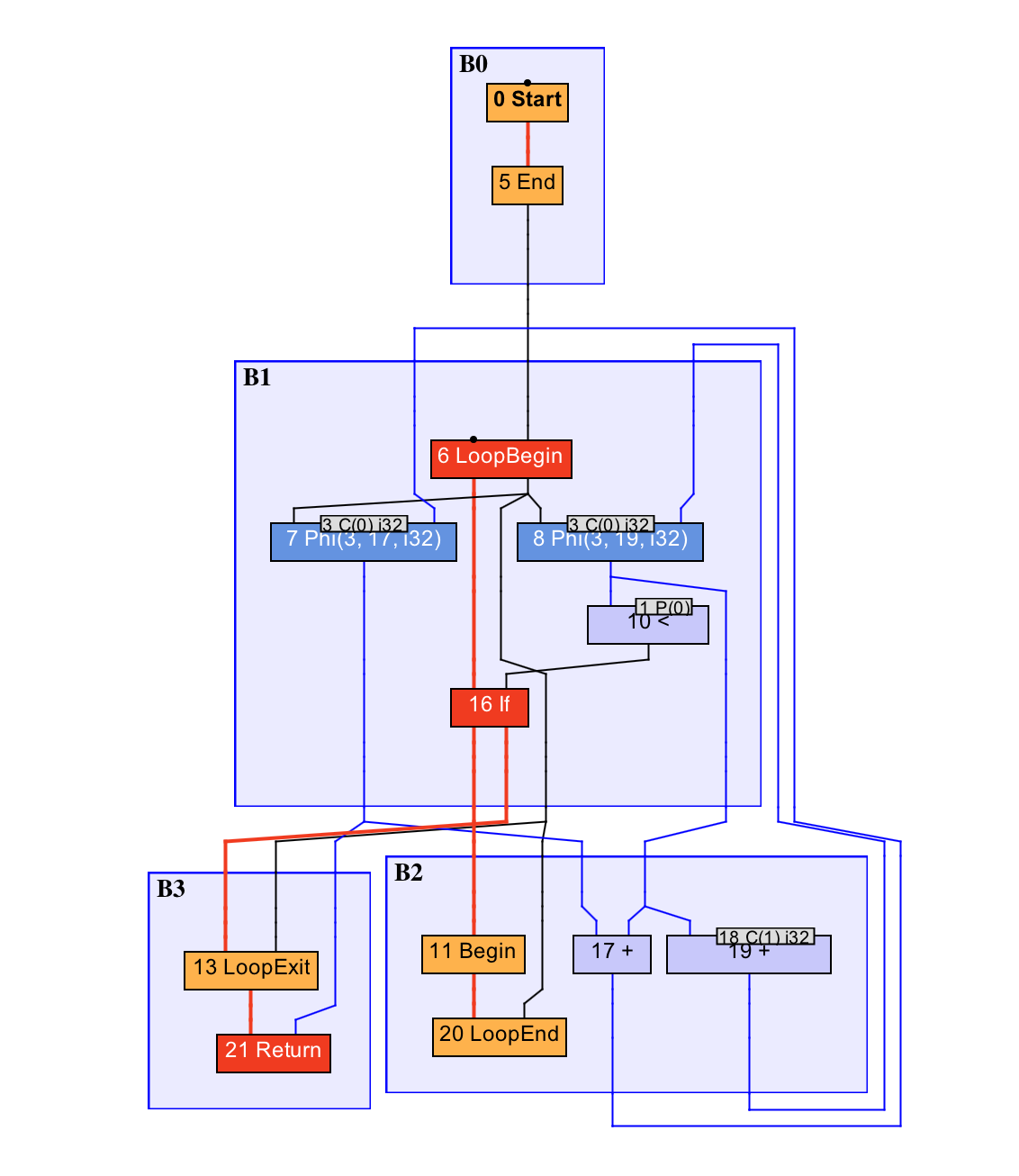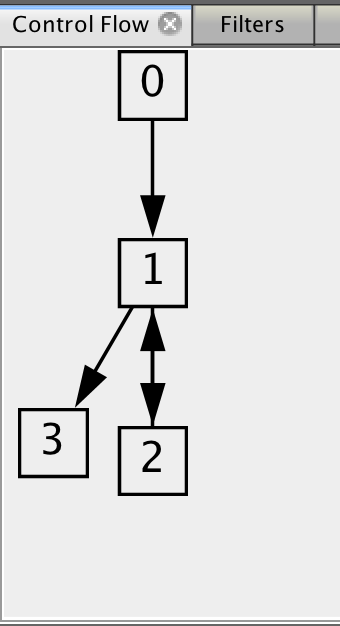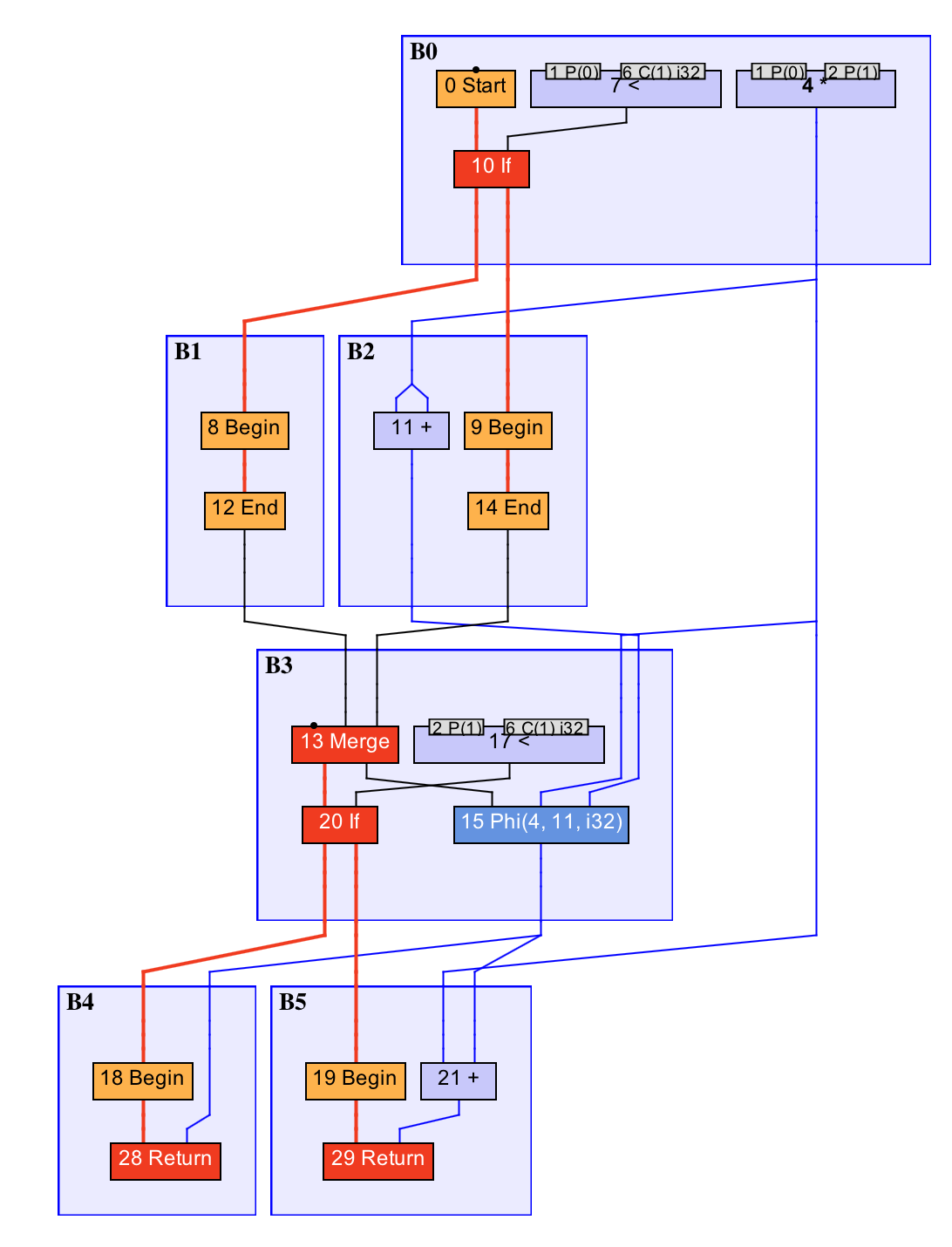18 即时编译器的中间表达形式

1. 中间表达形式（IR）

y = 1;
y = 2;
x = y;

y1 = 1;
y2 = 2;
x1 = y2;

x1=4*1024 经过常量折叠后变为 x1=4096
x1=4; y1=x1 经过常量传播后变为 x1=4; y1=4
y1=x1*3 经过强度削减后变为 y1=(x1<<1)+x1
if(2>1){y1=1;}else{y2=1;}经过死代码删除后变为 y1=1

SSA IR 会带来一个问题，那便是不同执行路径可能会对同一变量设置不同的值。例如下面这段代码 if 语句的两个分支中，变量 y 分别被赋值为 0 或 1，并且在接下来的代码中读取 y 的值。此时，根据不同的执行路径，所读取到的值也很有可能不同。

x = ..;
if (x > 0) {
y = 0;
} else {
y = 1;
}
x = y;

x1 = ..;
if (x1 > 0) {
y1 = 0;
} else {
y2 = 1;
}
y3 = Phi(y1, y2);
x2 = y3;

2. Sea-of-nodes

HotSpot 里的 C2 采用的是一种名为 Sea-of-Nodes 的 SSA IR。它的最大特点，便是去除了变量的概念，直接采用变量所指向的值，来进行运算。

Graal 的 IR 同样也是 Sea-of-Nodes 类型的，并且可以认为是 C2 IR 的精简版本。由于 Graal 的 IR 系统更加容易理解，而且工具支持相对来说也比较全、比较新，所以下面我将围绕着 Graal 的 IR 系统来讲解。

public static int foo(int count) {
int sum = 0;
for (int i = 0; i < count; i++) {
sum += i;
}
return sum;
}IR 图C2 的做法便是将这种时序上的先后记录为内存依赖，并让节点调度算法在进行调度时考虑这些内存依赖关系。Graal 则将内存读写转换成固定节点。由于固定节点存在先后关系，因此无须额外记录内存依赖。

3. Gloval Value Numbering

GVN 是一种发现并消除等价计算的优化技术。举例来说，如果一段程序中出现了多次操作数相同的乘法，那么即时编译器可以将这些乘法并为一个，从而降低输出机器码的大小。如果这些乘法出现在同一执行路径上，那么 GVN 还将省下冗余的乘法操作。

public static int foo(int a, int b) {
int sum = a * b;
if (a > 0) {
sum += a * b;
}
if (b > 0) {
sum += a * b;
}
return sum;
}总结与实践

// java -XX:+UnlockExperimentalVMOptions -XX:+UseJVMCICompiler -XX:CompileCommand='dontinline,CompilationTest::hash' -Dgraal.Dump=:3 -Dgraal.MethodFilter='CompilationTest.hash' -Dgraal.OptDeoptimizationGrouping=false CompilationTest
public class CompilationTest {
public static int hash(Object input) {
if (input instanceof Exception) {
return System.identityHashCode(input);
} else {
return input.hashCode();
}
}
public static void main(String[] args) throws InterruptedException {
for (int i = 0; i < 500000; i++) {
hash(i);
}